### Home > CALC > Chapter 6 > Lesson 6.3.2 > Problem6-92

6-92.
1. Consider the relation y3 − 3y = x3 + 1. Homework Help ✎

1. Find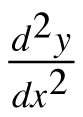in terms of x and y.

2. Find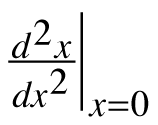3. Find all points where the derivative is undefined.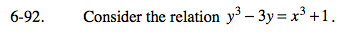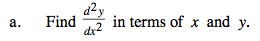$\text{Find } \frac{dy}{dx}:$

$\frac{d}{dx}(y^{3}-3y)=\frac{d}{dx}(x^{3}+1)$

$3y^{2}\frac{dy}{dx}-3\frac{dy}{dx}=3x^{2}\frac{dx}{dx}$

$\frac{dy}{dx}(3y^{2}-3)=3x^{2}$

$\frac{dy}{dx}=\frac{3x^{2}}{3y^{2}-3}=\frac{x^{2}}{y^{2}-1}$

$\text{Find } \frac{d^{2}y}{dx^{2}}:$

$\frac{dy}{dx}=\frac{x^{2}}{y^{2}-1}$

$\frac{d^{2}y}{dx^{2}}=\frac{2x\frac{dx}{dx}(y^{2}-1)-2y\frac{dy}{dx}(x^{2})}{(y^{2}-1)^{2}}=\frac{2x(y^{2}-1)-2y\frac{x^{2}}{y^{2}-1}(x^{2})}{(y^{2}-1)^{2}}$

You could simplify now, if you choose.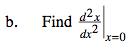$\text{Notice that } \frac{d^{2}y}{dx^{2}},\text{ which you found in part (a),}$

has both x and y variables.
So, before you evaluate, use the original equation
(y3 − 3y = x3 + 1) to find the y-value that corresponds with x = 0. It is possible that there will be more than one.

y3 − 3y = (0)3 + 1
y3 − 3y − 1 = 0
Use a calculator to find the THREE y-values that correspond with x = 0.

$\text{Evaluate } \frac{d^{2}y}{dx^{2}}\text{ at those THREE coordinate points.}$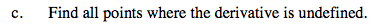$\text{The derivative, } \frac{dy}{dx} = \frac{x^2}{y^2-1},\text{ will be undefined where the denominator equals 0.}$